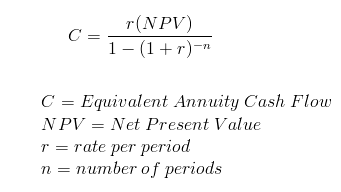# CFA Level II: Corporate Finance – Project Selection and Risks

#### Mutually Exclusive Projects with Unequal Lives

There are two methods we can use to pick projects when the project lives are different. Because the number of years is different, the project with the larger NPV is not necessarily better.

The replacement chain approach assumes that the shorter project will be repeated until its project life is equal to the life of the longer project. We would sum the NPVs of the number of times we repeat the project and compare that summed NPV to the NPV of the longer project.

Alternatively, there is the equivalent annual annuity approach, where we convert the NPVs of each project into an annual payment. The project with the higher annual payment has is the better value.

To compute the annual payment, input the values into a financial calculator. NPV is PV, FV is 0, N is the life of the project, and I is the WACC or cost of the project. We compute PMT.

The equation below will also solve for EAA:#### Risk Analysis

To estimate standalone risk of a capital investment or project, we can use sensitivity analysis, scenario analysis of Monte Carlo simulations.

Sensitivity analysis involves changing certain variables of a projects and recalculating the NPVs under these various changes. The variables could include sales, costs or WACCs. Projects that change more given a change in a variable are riskier.

Scenario Analysis involves calculating NPVs under various differing macro environments, like low sales, low price. Each environment is assigned a base case, worst case and best case and given probabilities. We then analyze the variance between the NPVs of each case.

Monte Carlo analysis uses assumed probability distributions for the variables of the NPV, and then select random values within the distributions for each variable. The NPVs are calculated many times, and we use the distribution of the NPVs to estimate an expected NPV. We can also use the standard deviation of the NPV as a measure of stand-alone risk for the project.